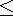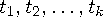Functions and CALL Routines

# DEPTAB Function

Returns the depreciation from specified tables.
 Category: Financial

## Syntax

 DEPTAB(p,v,t1,...,tn)

### Arguments

p

is numeric, the period for which the calculation is to be done. For noninteger p arguments, the depreciation is prorated between the two consecutive time periods that precede and follow the fractional period.

v

is numeric, the depreciable initial value of the asset.

t1,t2, . . . ,tn

are numeric, the fractions of depreciation for each time period with t1+t2+...tn1.

The DEPTAB function returns the depreciation by using specified tables. The formula isFor a given p, only the argumentsneed to be specified with k=ceil(p) .

An asset has a depreciable initial value of \$1,000 and a five-year lifetime. Using a table of the annual depreciation rates of .15, .22, .21, .21, and .21 during the first, second, third, fourth, and fifth years, respectively, the depreciation in the third year can be expressed asThe value that is returned is 210.00. The fourth rate, .21, and the fifth rate, .21, can be omitted because they are not needed in the calculation.Previous Page | Next Page | Top of Page